# Section A.3. CDMA-Based Cellular Systems

### A.3. CDMA-Based Cellular Systems

In CDMA, the uplink is generally the limiting link in terms of capacity . Consider N mobiles communicating to a single isolated base station. Assuming perfect power control, the signal from each mobile is received at the base station at its target power, S. Therefore, the interference from N 1 interfering mobile stations is

Equation A-21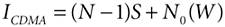Now the interference due to the UWB transmitters can be introduced to modify ICDMA to

Equation A-22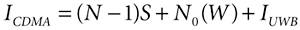The signal-to-interference ratio, SIR, for a particular user's signal arriving at the base station is

Equation A-23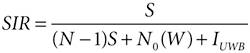The total interference power can be expressed as

Equation A-24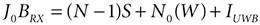where J0 is the combined PSD of the interference and receiver noise. The power, S, received from each mobile station is the product of the energy per bit, Eb, and the transmission bit rate, Rb:

Equation A-25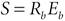Therefore, we can calculate Eb / J0 as

Equation A-26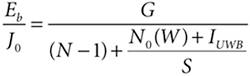where G is the processing gain, which is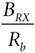Equation A-26 is based on the analysis of a single isolated cell. Due to the limited time scales associated with this study, factors such as imperfect power control, voice activity, and inter-cell interference have not been considered in this analysis.

Equation A-26 can be rearranged to calculate the target received power, S, which we now denote as SUWB:

Equation A-27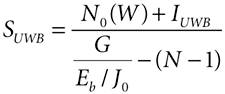A similar expression can be written for the target received power, SNO_UWB, when the CDMA base station receives no interference from UWB transmitters:

Equation A-28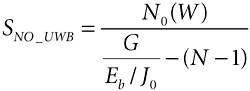The received powers, SUWB and SNO_UWB, required to maintain a target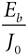can be calculated, and the UWB interference that makes SUWB greater than SNO_UWB by M dB can be evaluated. In this way the UWB PSD that increases the received power by a particular amount, M dB, can be identified. The increase in received power is

Equation A-29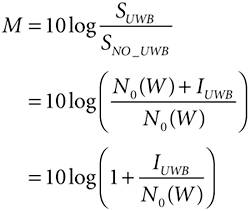Equations A-19 and A-29 can be combined to derive the UWB PSD, Gp, that increases the mobile station transmit power in a CDMA system by M dB:

Equation A-30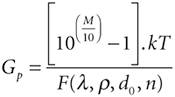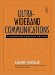Ultra-Wideband Communications: Fundamentals and Applications
ISBN: 0131463268
EAN: 2147483647
Year: 2005
Pages: 93

flylib.com © 2008-2017.
If you may any questions please contact us: flylib@qtcs.net